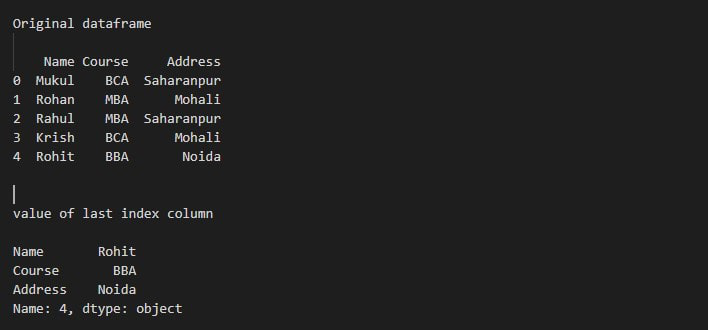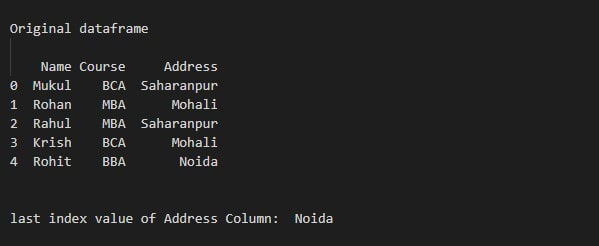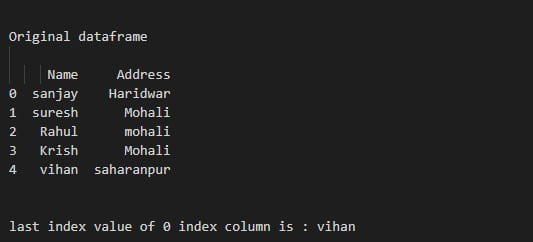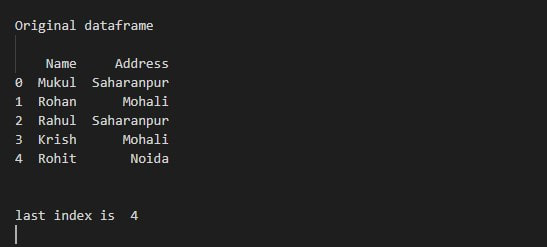# Access Index of Last Element in pandas DataFrame in Python

In this article, we are going to see how to access an index of the last element in the pandas Dataframe. To achieve this, we can use Dataframe.iloc, Dataframe.iget, and Dataframe.index. let’s go through all of them one by one.

Dataframe.iloc –  Pandas Dataframe.iloc is used to retrieve data by specifying its index. In python negative index starts from the end so we can access the last element of the dataframe by specifying its index to -1.

Syntax: pandas.DataFrame.iloc[]

Parameters:

Index Position: Index position of rows in integer or list of integer.

Return type: Data frame or Series depending on parameters

Example 1: The following program is to access the index of the last element from the entire Dataframe.

## Python3

 `# import pandas ` `import` `pandas as pd ` ` `  `# create dataframe ` `df ``=` `pd.DataFrame({``'Name'``: [``'Mukul'``, ``'Rohan'``, ``'Rahul'``,  ` `                            ``'Krish'``, ``'Rohit'``], ` `                   ``'Course'``: [``'BCA'``, ``'MBA'``, ``'MBA'``, ``'BCA'``, ` `                              ``'BBA'``], ` `                   ``'Address'``: [``'Saharanpur'``, ``'Mohali'``, ` `                               ``'Saharanpur'``, ``'Mohali'``,  ` `                               ``'Noida'``]}) ` ` `  `# Display original dataframe ` `print``(``"Original dataframe"``) ` `print``(df) ` ` `  `# Display last index value of dataframe ` `# iloc[-1] is return the last element of  ` `# all columns in DataFrame. ` `print``(``"value of last index column"``) ` `print``(df.iloc[``-``1``]) `

Output:Example 2:

The following program is to access the index of the last element from a specific column.

## Python3

 `# import pandas ` `import` `pandas as pd ` ` `  `# create dataframe ` `df ``=` `pd.DataFrame({``'Name'``: [``'Mukul'``, ``'Rohan'``, ``'Rahul'``, ` `                            ``'Krish'``, ``'Rohit'``], ` `                   ``'Course'``: [``'BCA'``, ``'MBA'``, ``'MBA'``,  ` `                              ``'BCA'``, ``'BBA'``], ` `                   ``'Address'``: [``'Saharanpur'``, ``'Mohali'``,  ` `                               ``'Saharanpur'``, ``'Mohali'``,  ` `                               ``'Noida'``]}) ` ` `  `# Display original dataframe ` `print``(``"Original dataframe"``) ` `print``(df) ` ` `  `# Display last index value of Address dataframe ` `print``(``"last index value of Address Column: "``, df[``'Address'``].iloc[``-``1``]) `

Output:Dataframe.iat() function – Pandas iat[] method is used to return data in a dataframe at the passed location. The passed location is in the format [position in the row, position in the column]. This method works similarly to Pandas iloc[] but iat[] is used to return only a single value and hence works faster than it.

Syntax: Dataframe.iat[row, column]

Parameters:

• position: Position of element in column
• label: Position of element in row

Return type: Single element at passed position

Example 3:

Under, this example we will be using the df.iat() function.

## Python3

 `# import pandas ` `import` `pandas as pd ` ` `  `# create dataframe ` `df ``=` `pd.DataFrame({``'Name'``: [``'sanjay'``, ``'suresh'``, ` `                            ``'Rahul'``, ``'Krish'``,  ` `                            ``'vihan'``], ` `                   ``'Address'``: [``'Haridwar'``, ``'Mohali'``,  ` `                               ``'mohali'``, ``'Mohali'``,  ` `                               ``'saharanpur'``]}) ` ` `  `# Display original dataframe ` `print``(``" Original dataframe "``) ` `print``(df) ` ` `  `# Display last index value of 0 index column ` `print``(``"last index value of 0 index column is "``, df.iat[``-``1``, ``0``]) `

Output:Example 4:

In this example, we are using the df.index() function to access the last element of the given data frame in python language.

## Python3

 `# import pandas ` `import` `pandas as pd ` ` `  `# create dataframe ` `df ``=` `pd.DataFrame({``'Name'``: [``'Mukul'``, ``'Rohan'``,  ` `                            ``'Rahul'``, ``'Krish'``, ` `                            ``'Rohit'``], ` `                   ``'Address'``: [``'Saharanpur'``, ``'Mohali'``,  ` `                               ``'Saharanpur'``, ``'Mohali'``,  ` `                               ``'Noida'``]}) ` ` `  `# Display original dataframe ` `print``(``" Original dataframe "``) ` `print``(df) ` ` `  `# Display last index value of dataframe ` `# iloc[-1] is return the last element of ` `# all columns in DataFrame. ` `print``(``" last index is "``, df.index[``-``1``]) `

Output:Whether you're preparing for your first job interview or aiming to upskill in this ever-evolving tech landscape, GeeksforGeeks Courses are your key to success. We provide top-quality content at affordable prices, all geared towards accelerating your growth in a time-bound manner. Join the millions we've already empowered, and we're here to do the same for you. Don't miss out - check it out now!

Previous
Next